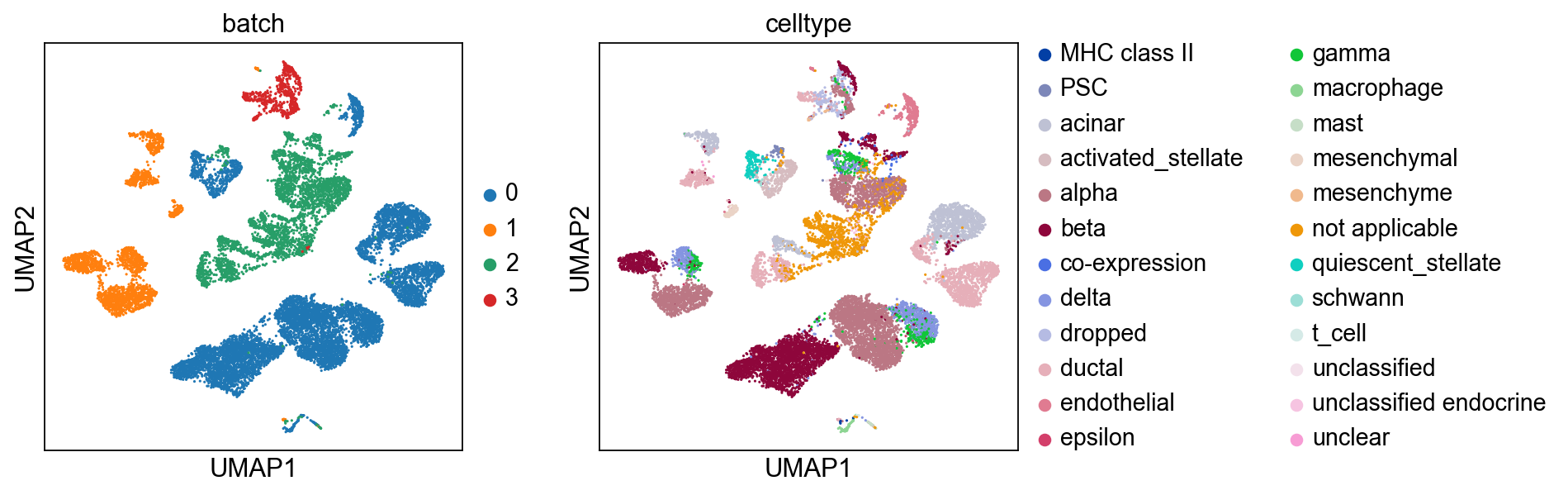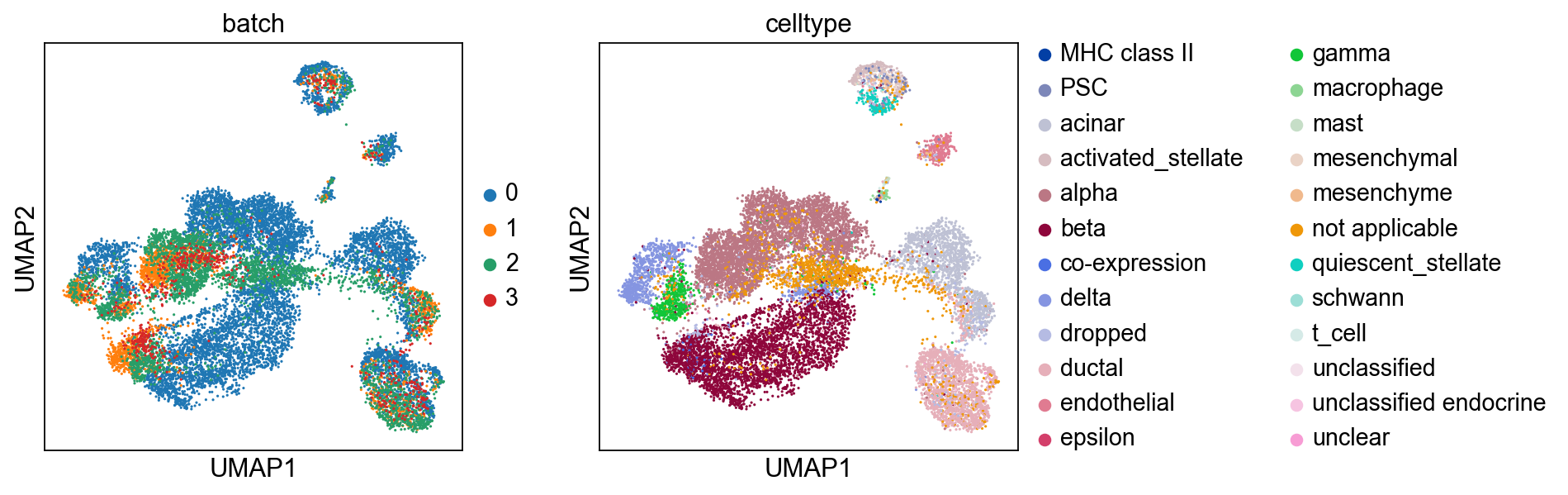In :
import scanpy as sc
import bbknn

In :
sc.settings.verbosity = 3             # verbosity: errors (0), warnings (1), info (2), hints (3)
sc.settings.set_figure_params(dpi=80)

scanpy==1.6.0 anndata==0.7.4 umap==0.4.6 numpy==1.18.1 scipy==1.4.1 pandas==1.0.1 scikit-learn==0.22.2.post1 statsmodels==0.11.1 python-igraph==0.8.2 leidenalg==0.8.3


Import some pancreas data, pooled from multiple experiments. The data features a heavy batch effect.

The object's creation is detailed here.

In :
adata = sc.read('pancreas.h5ad', backup_url='https://www.dropbox.com/s/qj1jlm9w10wmt0u/pancreas.h5ad?dl=1')

/usr/local/lib/python3.7/site-packages/anndata/compat/__init__.py:161: FutureWarning: Moving element from .uns['neighbors']['distances'] to .obsp['distances'].

This is where adjacency matrices should go now.
FutureWarning,
/usr/local/lib/python3.7/site-packages/anndata/compat/__init__.py:161: FutureWarning: Moving element from .uns['neighbors']['connectivities'] to .obsp['connectivities'].

This is where adjacency matrices should go now.
FutureWarning,Run BBKNN, which computes a batch balanced neighbourhood graph, which can then be used as the basis for a UMAP. This performs batch correction at the neighbourhood graph stage, and the resulting manifold has the experiments integrated better.

Running BBKNN requires a dimensionality reduction (PCA by default) present in .obsm of your object, and some sort of batch variable as a column in .obs. The documentation discusses all of the available parameters. This notebook shows the effect of some parameter choices on the data from In.

In :
bbknn.bbknn(adata, batch_key='batch')

computing batch balanced neighbors
finished: added to .uns['neighbors']
.obsp['distances'], distances for each pair of neighbors
.obsp['connectivities'], weighted adjacency matrix (0:00:04)
computing UMAPPark et al. 2020 improved batch mixing by introducing ridge regression into the workflow. Regressing out technical effect while including a biological grouping into the procedure is insufficient to remove batch effect from a UMAP based on a regular neighbour graph, but helps mix batches better when used as input for BBKNN. One may not be armed in a biological grouping at the time of analysis, in which case a coarse clustering (aiming to feature as many batches as possible per cluster) can be used in its place. This results in the following analysis flow:

bbknn
clustering
ridge regression
pca
bbknn



Let's generate and visualise a post-BBKNN clustering.

In :
sc.tl.leiden(adata, resolution=0.4)

running Leiden clustering